# The diagonal of rectangle problems

#### Number of problems found: 66

• Diagonal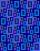Diagonal of the rectangle has length 46.4 cm. Angle between diagonal and londer sife of rectangle is 22°. Calculate area of the rectangle.
• RectangleIn a rectangle with sides, 8 and 9 mark the diagonal. What is the probability that a randomly selected point within the rectangle is closer to the diagonal than to any side of the rectangle?
• Perimeter and diagonal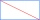The perimeter of the rectangle is 82 m, the length of its diagonal is 29 m. Find the dimensions of the rectangle.
• Diagonal of the rectangleCalculate the rectangle's diagonal, which area is 54 centimeters square, and the circuit is equal to 30 cm.
• Rectangle 3-4-5The sides of the rectangle are in a ratio of 3:4. The length of the rectangle diagonal is 20 cm. Calculate the content of the rectangle.
• Diagonal in rectangle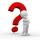In that rectangle ABCD is the center of BC point E and point F is center of CD. Prove that the lines AE and AF divide diagonal BD into three equal parts.
• RectangleThere is a rectangle with a length of 12 cm and a diagonal 8 cm longer than the width. Calculate the area of a rectangle.
• Diagonal to areaCalculate the area of a rectangle in which the length of the diagonal is 10 cm.
• Rectangle diagonalThe rectangle, one side of which is 5 cm long, is divided by a 13 cm diagonal into two triangles. Calculate the area of one of these triangles in cm2.
• RectangleThe length of the rectangle are in the ratio 5:12 and the circumference is 238 cm. Calculate the length of the diagonal and area of rectangle.
• DiagonalCalculate the diagonal length of the rectangle ABCD with sides a = 8 cm, b = 7 cm.
• Rectangle 35Find the area of a rectangle when the diagonal is equal to 30 cms and the width is double the length.
• Diagonal - simple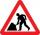Calculate the length of the diagonal of a rectangle with dimensions 5 cm and 12 cm.
• RectangleCalculate the length of the side GN and diagonal QN of rectangle QGNH when given: |HN| = 25 cm and angle ∠ QGH = 28 degrees.
• RectangleIn rectangle ABCD with sides, |AB|=19, |AD|=16 is from point A guided perpendicular to the diagonal BD, which intersects at point P. Determine the ratio ?.
• A rectangle 2A rectangle has a diagonal length of 74cm. Its side lengths are in ratio 5:3. Find its side lengths.
• RectangleThe rectangle has a perimeter 75 cm. Diagonal length is 32.5 cm. Determine the length of the sides.
• Area of a rectangleCalculate a rectangle area with a diagonal of u = 12.5cm and a width of b = 3.5cm. Use the Pythagorean theorem.
• Rectangle - desc circle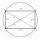Length of the sides of the rectangle are at a ratio 1: 3 . Radius of the circle circumscribed to rectangle is 10 cm. Calculate the rectangle's perimeter.
• Circumscription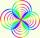Calculate radius of the Circumscribed circle in the rectangle with sides 20 and 19. It can be rectangle inscribed by circle?

Do you have an interesting mathematical word problem that you can't solve it? Submit a math problem, and we can try to solve it.

We will send a solution to your e-mail address. Solved examples are also published here. Please enter the e-mail correctly and check whether you don't have a full mailbox.

Please do not submit problems from current active competitions such as Mathematical Olympiad, correspondence seminars etc...
Diagonal - math problems. Rectangle Problems.